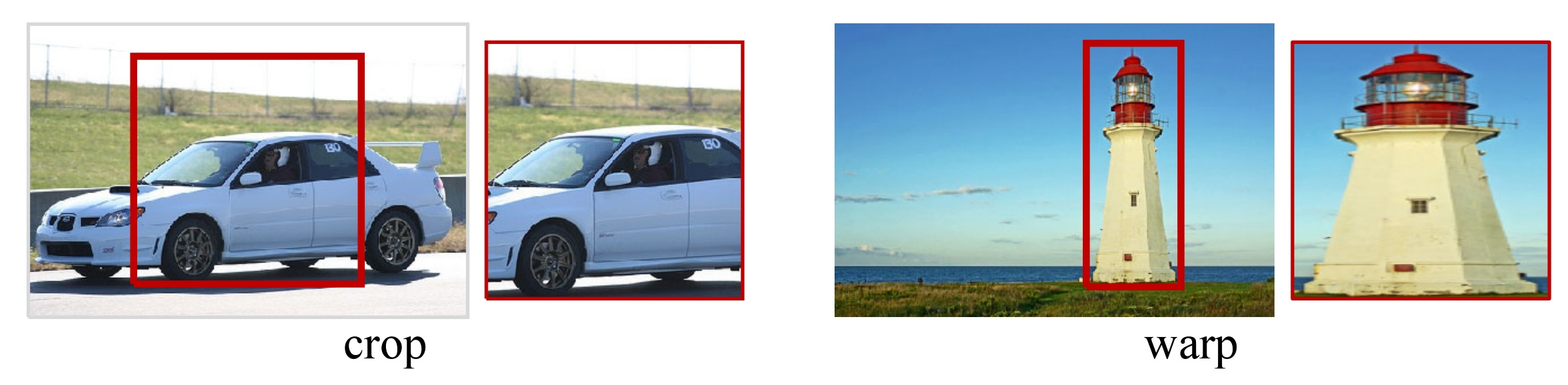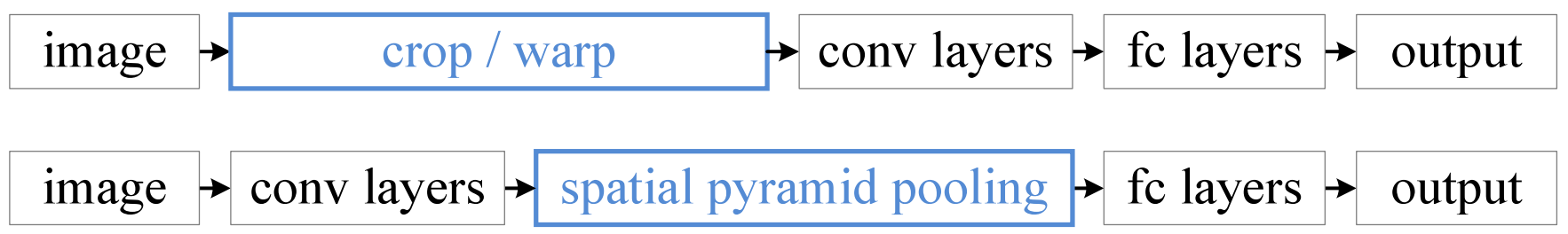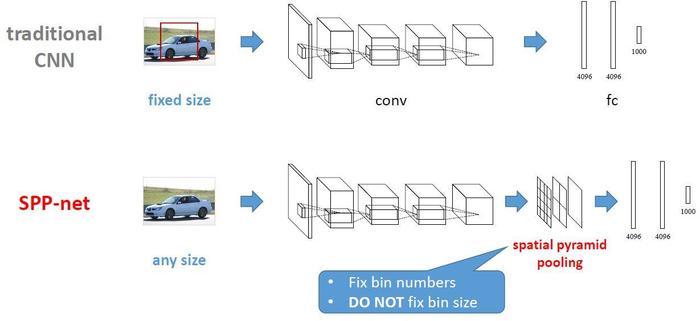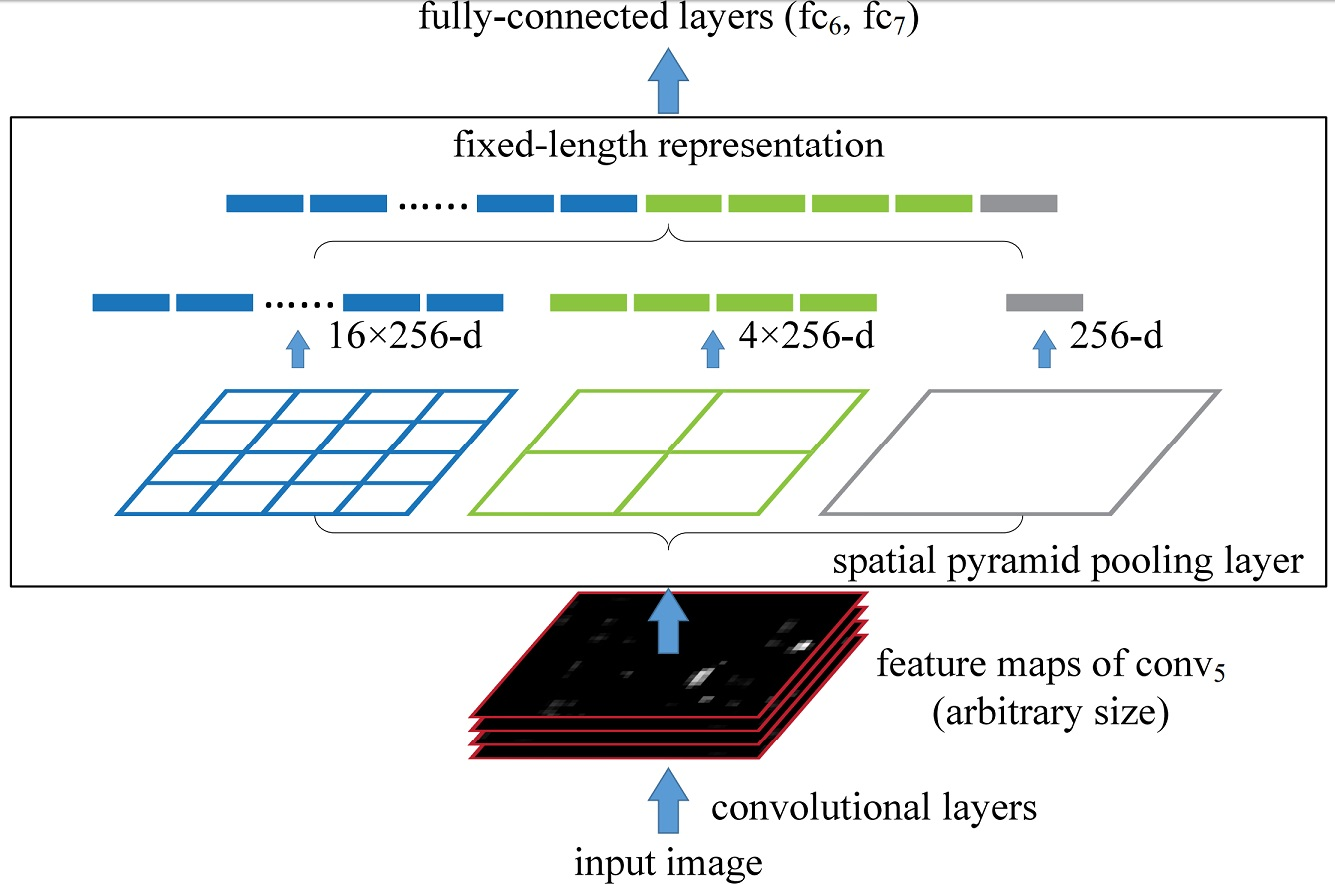# 使用 Spatial Pyramid Pooling 让 CNN 接受可变尺寸的图像

### 传统 CNN 的弊端### SPP-Net 概述SPP-Net 中有若干个并行的池化层，将卷积层的结果 $$[w\times h\times d]$$ 池化成 $$[1\times 1],[2\times 2], [4\times 4], \cdots$$ 的一层层结果，再将其所有结果与 FC 层相连。### SPP-Net 结构细节• 感受野大小 $$[w_r\times h_r]$$：$$w_r = \lceil\frac{w}{n}\rceil$$，$$h_r = \lceil\frac{h}{n}\rceil$$
• 步长 $$(s_w, s_h)$$：$$s_w = \lfloor\frac{w}{n}\rfloor$$，$$s_h = \lfloor\frac{h}{n}\rfloor$$

• 感受野大小应为 $$[\lceil\frac{30}{4}\rceil\times \lceil\frac{42}{4}\rceil] = [8\times 11]$$
• 步长应为 $$(\lfloor\frac{30}{4}\rfloor, \lfloor\frac{42}{4}\rfloor) = (7, 10)$$

### SPP-Net 训练方法

• Single-Size：将所有的图片固定到同一尺度。
• Multi-Size：将原图片通过 crop 得到某一尺度 A，再把 A 通过 warp 放缩成更小的尺寸 B。之后用 A 尺度训练一个 epoch，再用 B 尺度训练一个 epoch，交替迭代。

### 在 pytorch 框架中实现 SPP

class SpatialPyramidPool2D(nn.Module):
"""
Args:
out_side (tuple): Length of side in the pooling results of each pyramid layer.

Inputs:
- input: the input Tensor to invert ([batch, channel, width, height])
"""
def __init__(self, out_side):
super(SpatialPyramidPool2D, self).__init__()
self.out_side = out_side

def forward(self, x):
out = None
for n in self.out_side:
w_r, h_r = map(lambda s: math.ceil(s/n), x.size()[2:])    # Receptive Field Size
s_w, s_h = map(lambda s: math.floor(s/n), x.size()[2:])   # Stride
max_pool = nn.MaxPool2d(kernel_size=(w_r, h_r), stride=(s_w, s_h))
y = max_pool(x)
if out is None:
out = y.view(y.size(), -1)
else:
out = torch.cat((out, y.view(y.size(), -1)), 1)
return out

nn.Sequential(
nn.Conv2d(
in_channels=1,
out_channels=16,
kernel_size=5,
stride=1,
)